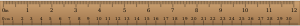# Definition of InchAn inch is an Imperial unit for measuring length.

A common tool for estimating the length of an inch is to use the length from the top knuckle of your thumb up to the tip of your thumb. Of course, this length is different in everybody, so it isn't a very accurate (or standard) estimate.

There are $12$ inches in a foot and $3$ feet ($36$ inches) in a yard.

The abbreviation for inches is in, or the double quotation mark, ". For example, you could write $23$ inches as $23 \text{ in}$ or $23\!"$.

So that you can convert for your grandparents or American friends, one inch is equal to $2.54$ centimetres.

### Description

The aim of this dictionary is to provide definitions to common mathematical terms. Students learn a new math skill every week at school, sometimes just before they start a new skill, if they want to look at what a specific term means, this is where this dictionary will become handy and a go-to guide for a student.

### Audience

Year 1 to Year 12 students

### Learning Objectives

Learn common math terms starting with letter I

Author: Subject Coach
You must be logged in as Student to ask a Question.